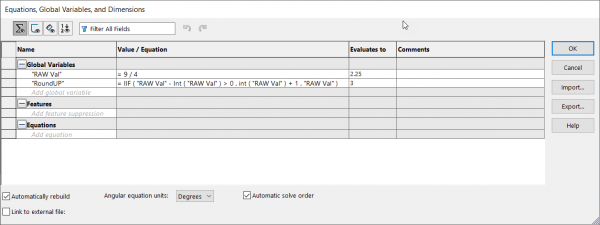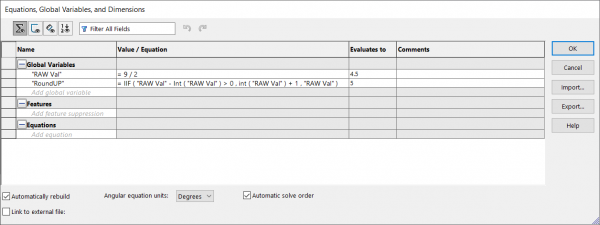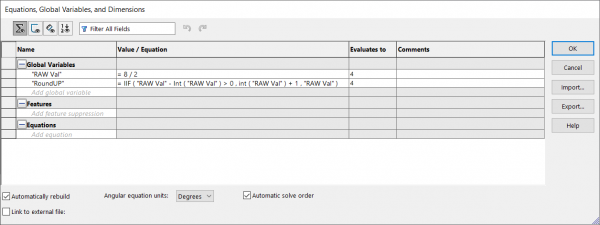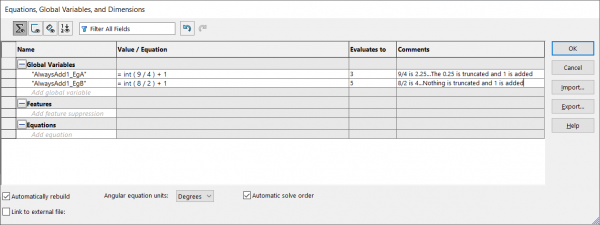# Rounding Up in SOLIDWORKS EquationsArticle by Prasadh Annalingam, CSWE updated October 16, 2020

###### Article

Similar to other programs such as Microsoft Excel, SOLIDWORKS has functions available to round numbers. However, certain workflow might require you to always Round Down or Up regardless of the decimal value.

Before we get started, if you are interested in SOLIDWORKS rounding in general or how to Round Down, please click the following link: How To Round Numbers in SOLIDWORKS Equations. If you don’t feel like clicking that link, just know that SOLIDWORKS uses a “To Even Rounding Method” also known as “Round Half to Even”. So the 5 after the decimal place will be rounded either up or down, whichever will result in an even integer (3.5 and 4.5 both round to 4).

## SOLIDWORKS Equations Scenario

We need to order stock material from your vendor and can only put in orders in full feet measurements. We calculate how much we need for our project with SOLIDWORKS Equations and it comes up to 3.2 feet. Thus, we will need 4 feet of material purchased.

### Rounding SOLIDWORKS Equations

Have SOLIDWORKS Equations show us the amount we need by always Rounding Up. So the big questions is…how can we always Round Up? Unfortunately, there is no simple way to Round Up in SOLIDWORKS, as of yet at least. What we can do is use an additional equation as a workaround to always Round Up. First we will find the raw value with decimals. The second equation (using an will round up/down if the integer part of the number is odd/even respectively. See the next three images for examples.Round Up 9/4Round Up 9/2Round Up 8/2

```Equations Used:
RAW Val =  any value or equation required to be rounded up
RoundUp  = IIF ( "RAW Val" - Int ( "RAW Val" ) > 0 , int ( "RAW Val" ) + 1 , "RAW Val" )```

With the equation used above, a whole number will not round up (5.0 will remain 5.0, as intended). If you want to increase whole numbers by 1 as well, you can truncate all decimal values of the number by using int() and then simply adding 1. See examples below.Not every company has the same workflow or practices and so, we must be able to adapt and find solutions accordingly. The workaround mentioned in this blog article is a way to always ensure your calculation is rounded up within SOLIDWORKS. See more SOLIDWORKS Equation articles for techniques that can help you work much more efficiently.